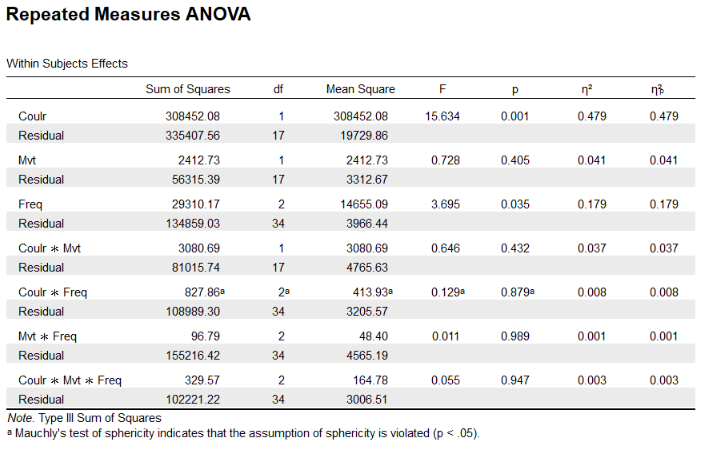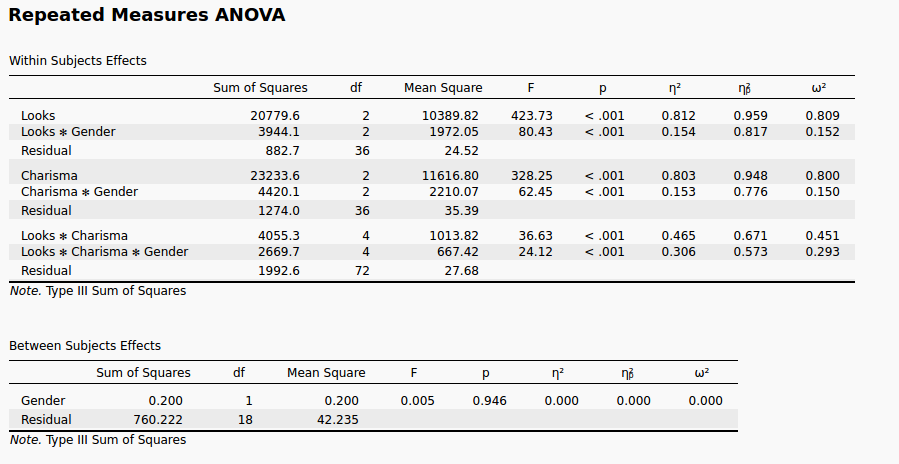#### Howdy, Stranger!

It looks like you're new here. If you want to get involved, click one of these buttons!

Supported by

# similar eta-square and partial eta-square

Hi !
I'm new to JASP and I ran an ANOVA with 12 factors. I obtain for each effect a partial eta-square similar to the eta-square. That should not happen, what am I doing wrong ?Thank you !
Raph

• Hmm. Let me ask a team member and get back to you.
E.J.

• OK, based on some quick research by Johnny van Doorn, it appears that the formula is in need of correction when there are within-subject factors. This is a good catch. We'll fix this in our upcoming release.
Cheers,
E.J.

• edited July 2017

Well, glad I could help !
I've done my η² by hand and I believe partial η² are correct, the error seems to come from the η² computation (Levine & Hullett 02).
Can you guys keep this thread posted when this is fixed ?
cheers,
Raph

• Yes, Johnny will look into it when he returns from a conference and we'll update you on our progress.
E.J.

• Hi Raph,
Thank you for bringing this to our attention, and my apologies for the tardy reply. After reading up on effect sizes for within subjects designs, and consulting some of the true statistics guru's at our department, I have come to the conclusion that the eta squared as reported does not differ from the partial eta squared when dealing with a design with only within subjects factors. If you were to add a between subjects factor, the partial eta does differ from the nonpartial eta.
This has to do with the formula used to compute the two effect sizes. For the nonpartial eta squared, the sum of squares of the effect is divided by the total sum of squares, whereas the denominator for the partial eta squared is the error term of only the effect. If you were to have only one factor, this means that the partial eta squared and nonpartial eta squared does not differ, as the error term of the effect constitutes the total error term. This is also how it is described in the article you linked.
The tricky thing with within subjects factors is the way the total error term is calculated. JASP (and SPSS for that matter) gives separate total error terms for each within subjects factor. It is not possible to sum these error terms (for a "total total error term"), because that would result in errors being counted double (or triple if you have three-way design) as a result of the within subjects design and would therefore provide a distorted image of the fit.
If attached a little example from the Andy Field book to illustrate the way these total error terms are made up. Here we have two within subjects factors (Looks and Charisma, both with three levels) and one between subjects factor (Gender). In this example, the partial and nonpartial eta squared do differ from each other, as there is a between subjects factor. So the denominator for calculating the eta squared for Looks is 20779.6 + 3944.1 + 882.7 = 25606.4, or the total error term (looking only within the within subjects factor of Looks). For the partial eta squared, the sum of squares from the interaction is not counted anymore, so the denominator there is equal to 20779.6 + 882.7 = 21662.3, or the error term of the effect only. In both cases the numerator is equal to 20779.6, yielding the provided effect sizes. If I were to remove the factor Gender from the model, both of the denominators would be equal and yield the same result for eta squared and partial eta squared.
So in short, the similarity of the effect sizes in your case is due to having only within subjects factors, and because of the way the total sum of squares are calculated in that case (i.e., only total within the within subjects factors), the partial and nonpartial eta squared yield the same result.
I hope this clarifies your query. I do have to say it's a bit of a niche issue, and many textbooks I consulted shy away from discussing this topic, so if you have any more questions I'd be happy to help you sort those out!
Kind regards,
Johnny• Hi. I have a question about the eta squared when conducting a mixed design ANOVA. When conducting a mixed design ANOVA (2 within and 1 between factors) JASP and JAMOVI give me the same p values and the same partial eta squared. However, JAMOVI and JASP give me different eta squared. While JASP gives me a n² number very close to the np², JAMOVI gives a n² very different from the np². (e.g. np² = .138, JAMOVI gives me n²=.21 while JASP n²=.136).

I know that devs here are not responsible for the JAMOVI outputs. But I would like to ask if someone can tell me why these eta squared are so different.

Cheers.

• Hi. I have a question about the eta squared when conducting a mixed design ANOVA. When conducting a mixed design ANOVA (2 within and 1 between factors) JASP and JAMOVI give me the same p values and the same partial eta squared. However, JAMOVI and JASP give me different eta squared. While JASP gives me a n² number very close to the np², JAMOVI gives a n² very different from the np². (e.g. np² = .138, JAMOVI gives me n²=.21 while JASP n²=.136).

I know that devs here are not responsible for the JAMOVI outputs. But I would like to ask if someone can tell me why these eta squared are so different.

Cheers.

• Dear Gabriel,

Thanks for bringing this to our attention. We will look into it and keep you posted!

Cheers,

E.J.

• Hi Gabriel,

We use the approach I outlined in my previous post - you can read more about it here.

I am not sure what JAMOVI uses - maybe you can contact them on their github page.

One thing that could cause a discrepancy is that different types of sum of squares were used to obtain your results - this leads to different values for the effect sizes. However you do say the p-values and partial effect sizes are the same so that is probably not the case.

Cheers

Johnny

• Hi.

Thanks for the replies!

Cheers.